Function Repository Resource:

# CrossValidateModel

Check the quality of a data fitting model by splitting the data into test and validation sets multiple times

Contributed by: Sjoerd Smit
 ResourceFunction["CrossValidateModel"][data,fitfun] splits data into training and validation sets multiple times in different ways, then applies fitfun to the training data and tests the result using the validation set. ResourceFunction["CrossValidateModel"][data,dist] performs cross-validation by using EstimatedDistribution to fit distribution dist to data. ResourceFunction["CrossValidateModel"][data,<|lbl1 → fitfun1,lbl2 → fitfun2,…|>] cross-validates all listed models, using the same set of training-validation splits for each model. ResourceFunction["CrossValidateModel"][data,{dist1,dist2,…}] performs cross-validation on multiple distributions by fitting them with EstimatedDistribution.

## Details and Options

The recognized formats for data are listed in the left-hand column below. The right-hand column shows examples of symbols that take the same data format. Note that Dataset is currently not supported.
 List: {…} Rule of lists: {…}→ {…} Association of lists: <|key1→ {…},…|> NetTrain
ResourceFunction["CrossValidateModel"] divides the data repeatedly into randomly chosen training and validation sets. Each time, fitfun is applied to the training set. The returned model is then validated against the validation set. The returned result is of the form {<|"FittedModel" model1, "ValidationResult" result1|>, }.
For any distribution that satisfies DistributionParameterQ, the syntax for fitting distributions is the same as for EstimatedDistribution, which is the function that is being used internally to fit the data. The returned values of EstimatedDistribution are found under the "FittedModel" keys of the output. The "ValidationResult" lists the average negative LogLikelihood on the validation set, which is the default loss function for distribution fits.
If the user does not specify a validation function explicitly using the "ValidationFunction" option, ResourceFunction["CrossValidateModel"] tries to automatically apply a sensible validation loss metric for results returned by fitfun (see the next table). If no usable validation method is found, the validation set itself will be returned so that the user can perform their own validation afterward.
The following table lists the types of models that are recognized, the functions that produce such models and the default loss function applied to that type of model:
 Any distribution that satisfies DistributionParameterQ EstimatedDistribution negative LogLikelihood LearnedDistribution LearnDistribution negative LogLikelihood RootMeanSquare of residuals PredictorFunction Predict PredictorMeasurementsObject (generated by PredictorMeasurements) ClassifierFunction Classify ClassifierMeasurementsObject (generated by ClassifierMeasurements) NetTrainResultsObject NetTrain[net,data,All, …] validation loss SpatialEstimatorFunction SpatialEstimate negative LogLikelihood
An explicit validation function can be provided with the "ValidationFunction" option. This function takes the fit result as a first argument and a validation set as a second argument. If multiple models are specified as an Association in the second argument of ResourceFunction["CrossValidateModel"], different validation functions for each model can be specified by passing an Association to the "ValidationFunction" option.
The Method option can be used to configure how the training and validation sets are generated. The following types of sampling are supported:
 "KFold" (default) splits the dataset into k subsets (default: k=5) and trains the model k times, using each partition as validation set once "LeaveOneOut" fit the data as many times as there are elements in the dataset, using each element for validation once "RandomSubSampling" split the dataset randomly into training and validation sets (default: 80% / 20%) repeatedly (default: five times) or define a custom sampling function "BootStrap" use bootstrap samples (generated with RandomChoice) to fit the model repeatedly without validation
The default Method setting uses k-fold validation with five folds. This means that the dataset is randomly split into five partitions, where each is used as the validation set once. This means that the data gets trained five times on 4/5 of the dataset and then tested on the remaining 1/5. The "KFold" method has two sub-options:
 "Folds" 5 number of partitions in which to split the dataset "Runs" 1 number of times to perform k-fold validation (each time with a new random partitioning of the data)
The "LeaveOneOut" method, also known as the jackknife method, is essentially k-fold validation where the number of folds is equal to the number of data points. Since it can be quite computationally expensive, it is usually a good idea to use parallelization with this method. It does have the "Runs" sub-option like the "KFold" method, but for deterministic fitting procedures like EstimatedDistribution and LinearModelFit, there is no value in performing more than one run since each run will yield the exact same results (up to a random permutation).

The method "RandomSubSampling" splits the dataset into training/validation sets randomly and has the following sub-options:

 "Runs" 1 number of times to resample the data into training/validation sets ValidationSet Scaled[1/5] number of samples to use for validation. When specified as Scaled[f], a fraction f of the dataset will be used for validation "SamplingFunction" Automatic function that specifies how to sub-sample the data
For the option "SamplingFunction", the function fun[nData,nVal] should return an Association with the keys "TrainingSet" and "ValidationSet". Each key should contain a list of integers that indicate the indices in the dataset.
The default sampling function corresponding to Automatic is Function[{nData,nVal},AssociationThread[{"TrainingSet","ValidationSet"},TakeDrop[RandomSample[Range[nData]],nData-nVal]]].
Bootstrap sampling is useful to get a sense of the range of possible models that can be fitted to the data. In a bootstrap sample, the original dataset is sampled with replacement (using RandomChoice), so the bootstrap samples can be larger than the original dataset. No validation sets will be generated when using bootstrap sampling. The following sub-options are supported:
 "Runs" 5 number of bootstrap samples generated "BootStrapSize" Scaled number of elements to generate in each bootstrap sample; when specified as Scaled[f], a fraction f of the dataset will be used
The "ValidationFunction" option can be used to specify a custom function that gets applied to the fit result and the validation data.
The "ParallelQ" option can be used to parallelize the computation using ParallelTable. Sub-options for ParallelTable can be specified as "ParallelQ"{True,opts}.

## Examples

### Basic Examples (5)

Generate some data and visualize it:

 In:=Out=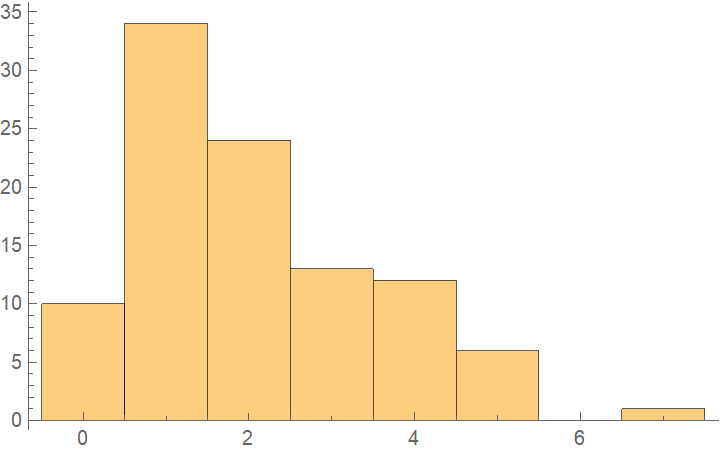Cross-validate the data by fitting a PoissonDistribution to five different training/validation splits of the data:

 In:=Out=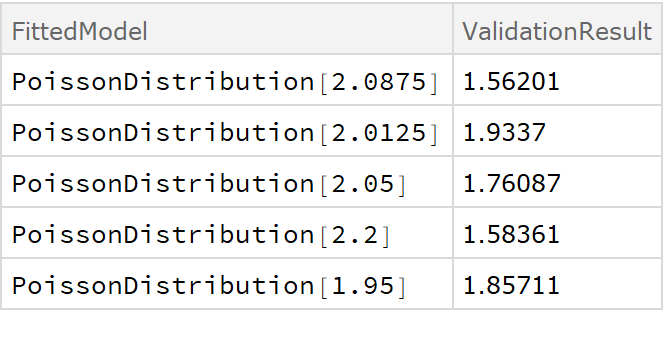The fitted values of λ can be extracted using Part:

 In:=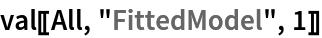Out=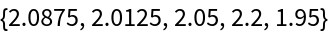Show the spread of the obtained values of λ and negative LogLikelihoods that were found:

 In:=Out=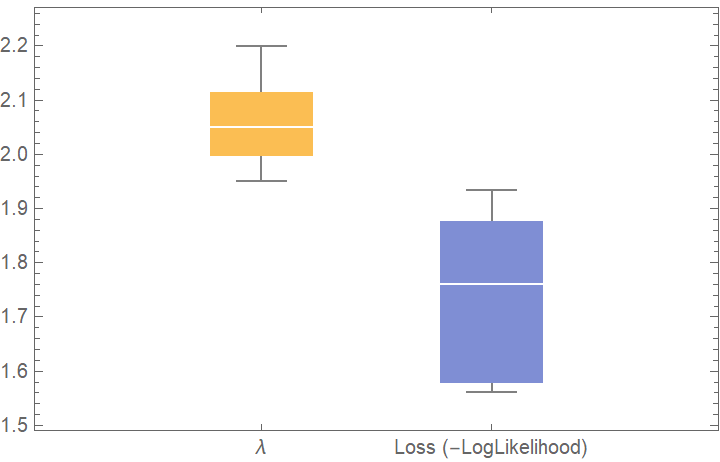Compare with a fit by another distribution. A lower negative log-likelihood indicates a better fit:

 In:=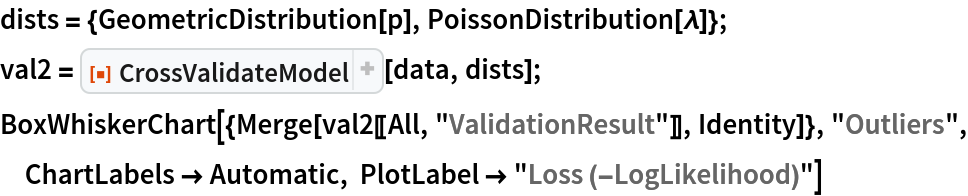Out=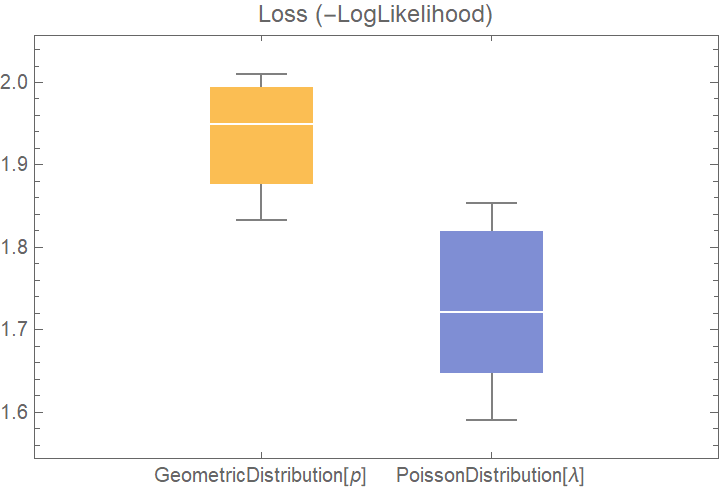### Scope (5)

CrossValidateModel works with LearnDistribution:

 In:=Out=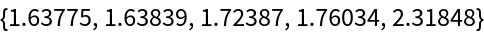CrossValidateModel works with LinearModelFit, GeneralizedLinearModelFit and NonlinearModelFit. For fit models that return a FittedModel object, CrossValidateModel will calculate the RootMeanSquare of the residuals of the fit:

 In:=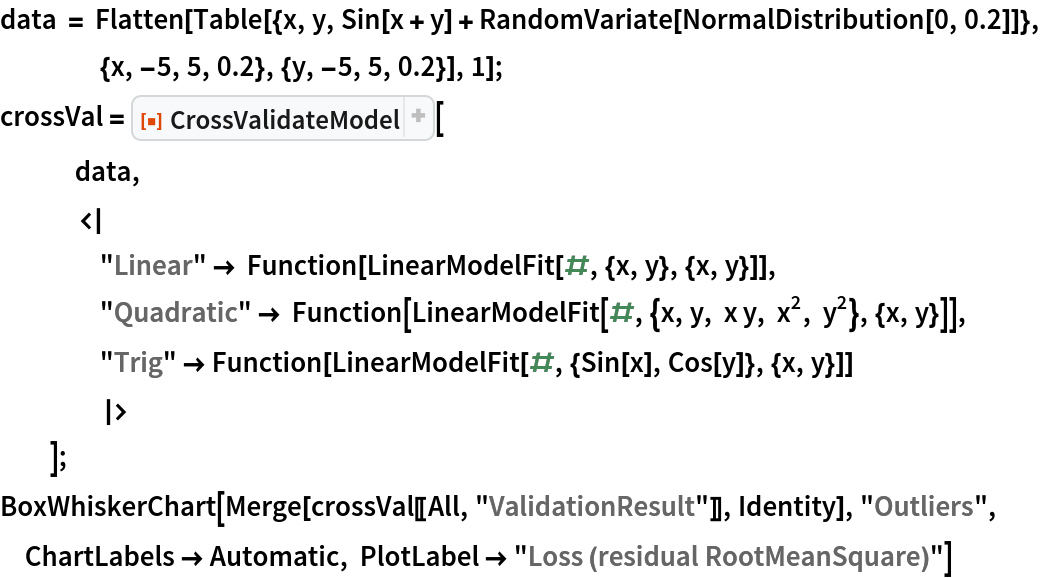Out=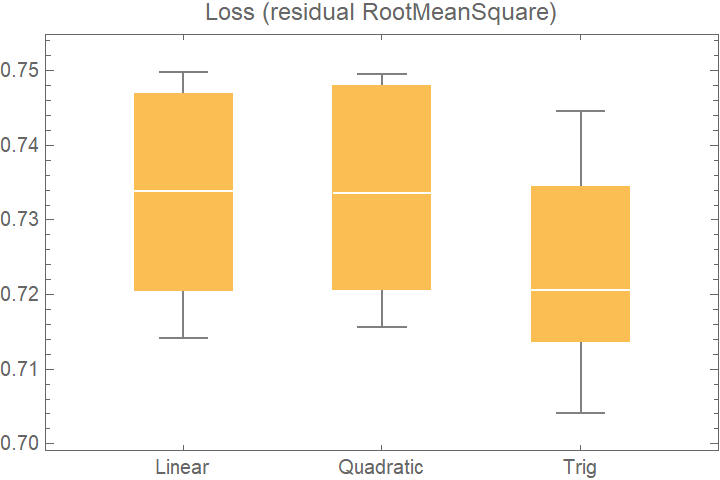CrossValidateModel also works with Fit if you specify that the returned model is a Function:

 In:=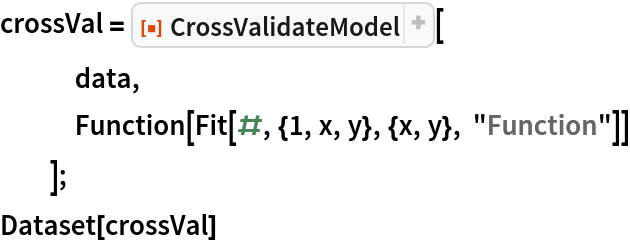Out=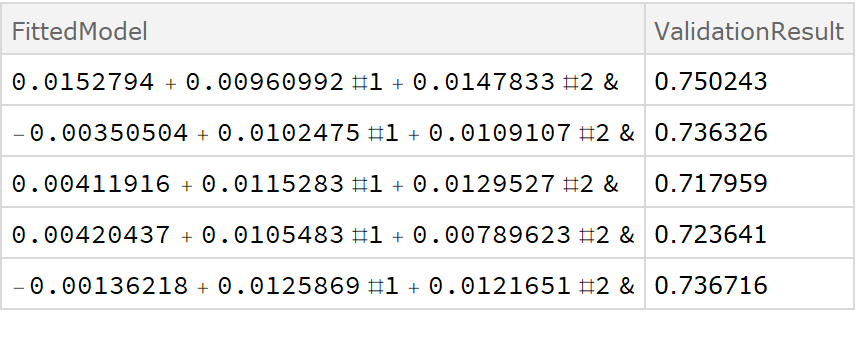To use the parameter rules generated by FindFit with the default validation option, it is necessary to specify the option "ValidationFunction"{Automatic,{fitExpr,{var1,var2,}},} to parse the fit expression and the independent variables into the default validation function:

 In:=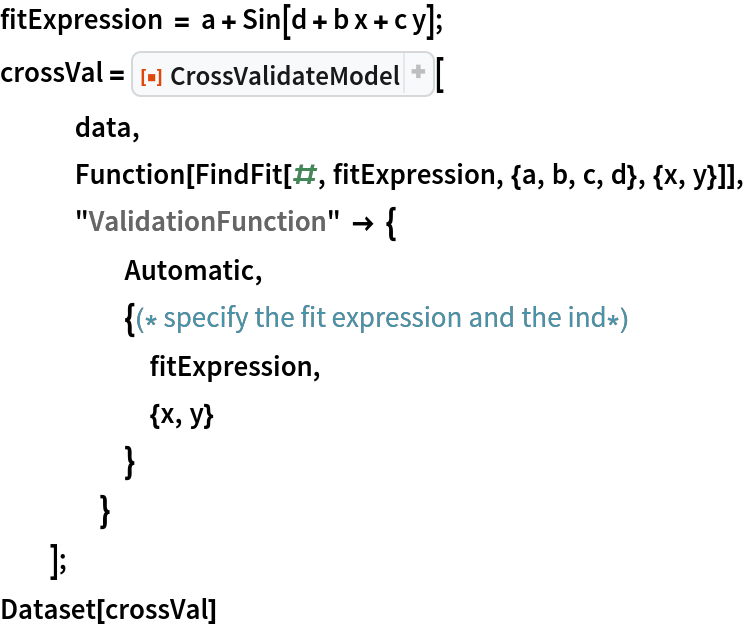Out=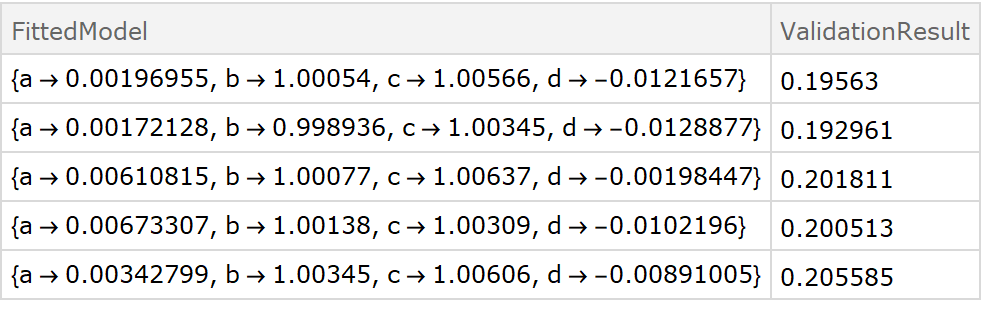When the fitting function uses Predict or Classify, the validation will be done with PredictorMeasurements or ClassifierMeasurements:

 In:=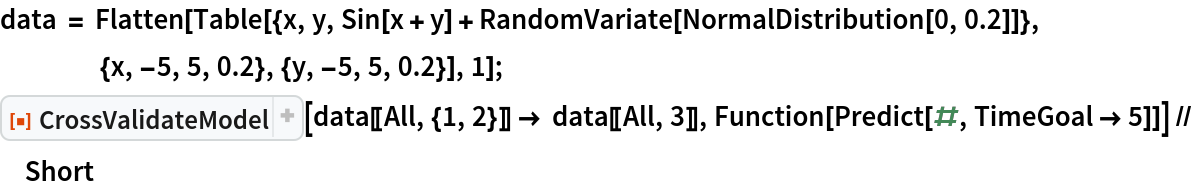Out=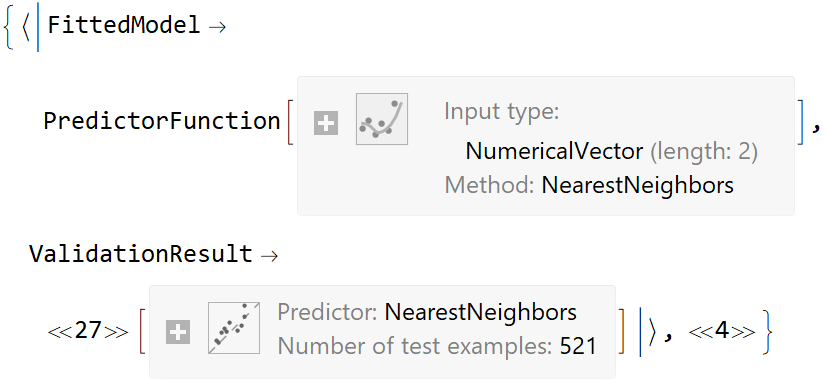In:=Out=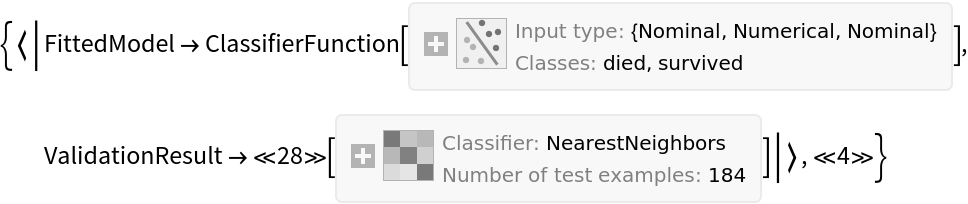CrossValidateModel can also be used with NetTrain. First define a network and pre-train it:

 In:=Out=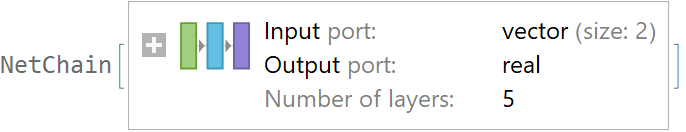Perform the cross validation. It is recommended to make sure that NetTrain returns a NetTrainResultsObject by using All as the third argument. The returned "ValidationResult" is the average loss of the trained network evaluated on the validation set:

 In:=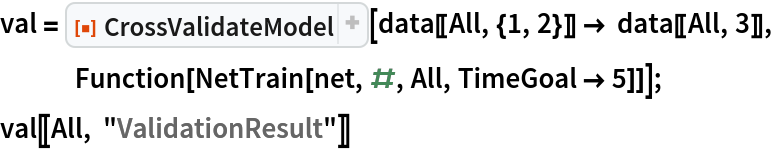Out=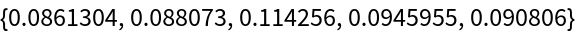SpatialEstimate is supported:

 In:=Out=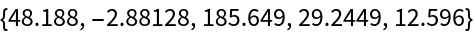SpatialEstimate works on GeoPosition arrays:

 In:=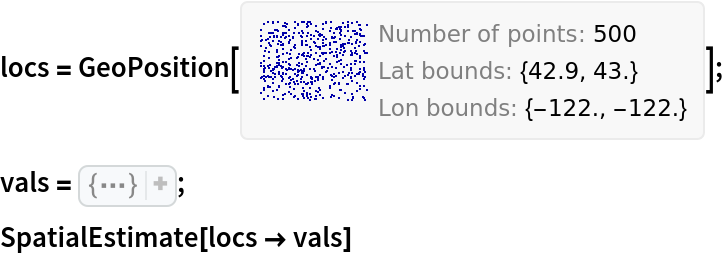Out=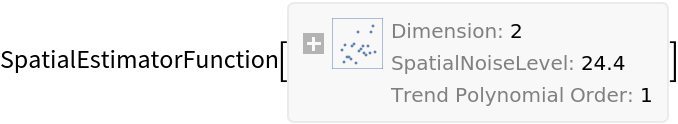But to do cross validation on GeoPosition arrays, it's necessary to unpack the array so that it can be sampled:

 In:=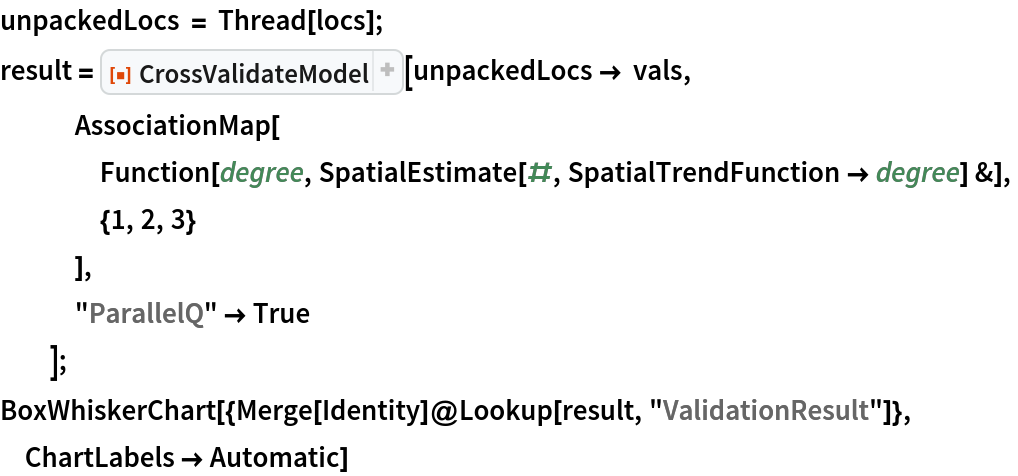Out=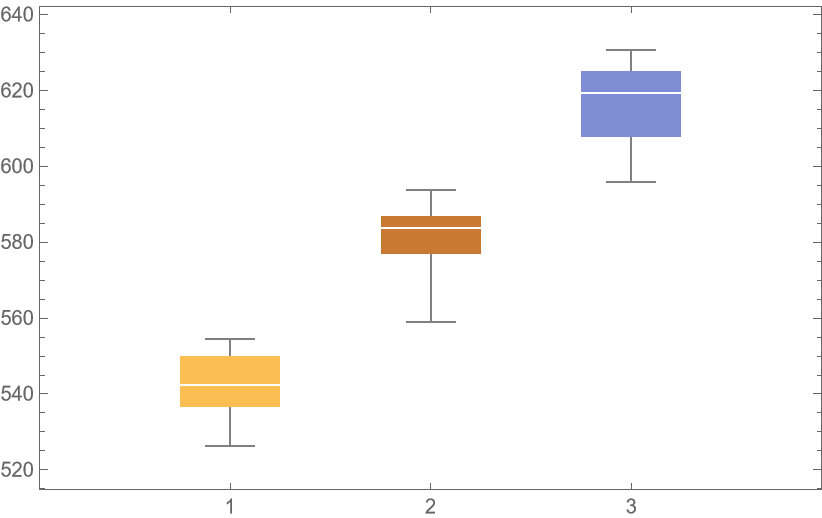### Options (11)

#### Method (4)

Suboptions "Folds" and "Runs" are support for the "KFold" method. Note how the total number of model fits is equal to "Folds" × "Runs":

 In:=Out=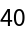Here is an example input using the "LeaveOneOut" method:

 In:=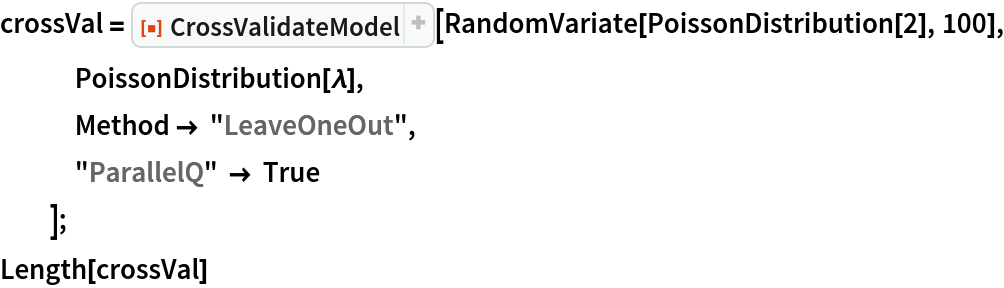Out=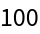Use the "RandomSubSampling" method. The "SamplingFunction" is set in such a way that it is equivalent to the default option "SamplingFunction" Automatic:

 In:=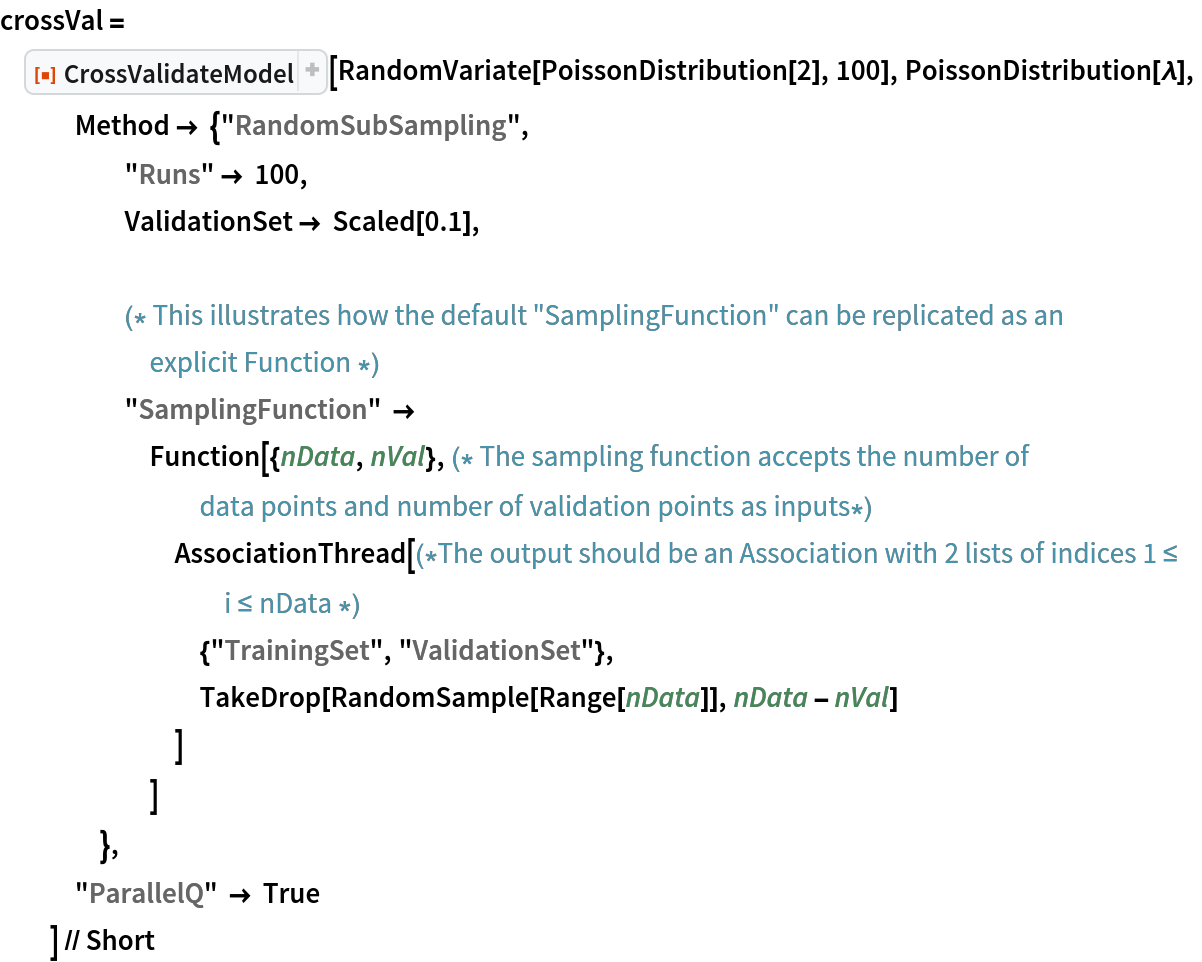Out=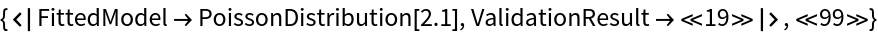In this example, we use bootstrap sampling to estimate the error bars on the value of λ:

 In:=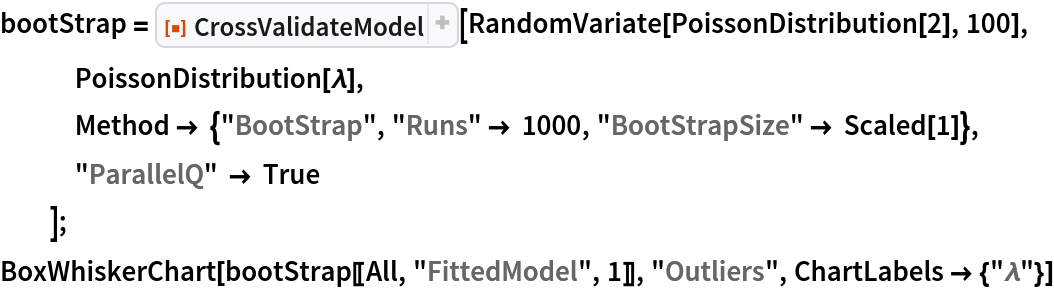Out=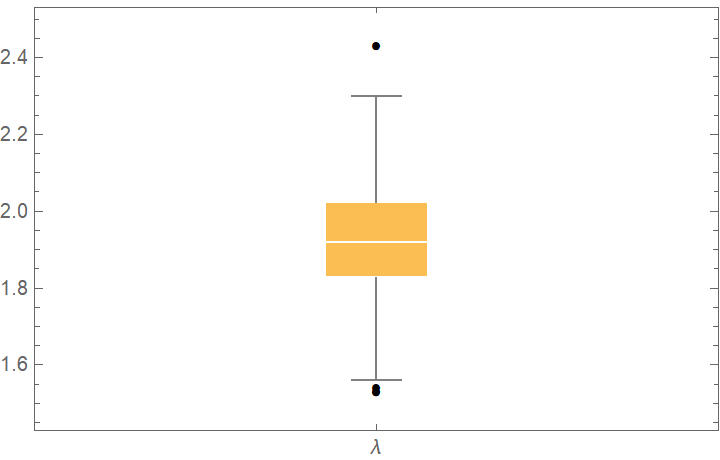#### ValidationFunction (6)

Specify a function that calculates the CDF fitted distribution at the points given by the validation data. We know that if the fitted distribution is the true distribution, then the obtained points should be distributed as UniformDistribution[0, 1], as can be illustrated with TransformedDistribution:

 In:=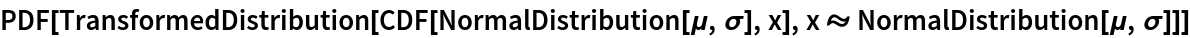Out=By calculating the CDF of the validation data, we can combine the results and use a QuantilePlot to judge if the validation data follows the same distribution as the fit. The quantile plot suggests that the ExponentialDistribution gives the best fit (as expected):

 In:=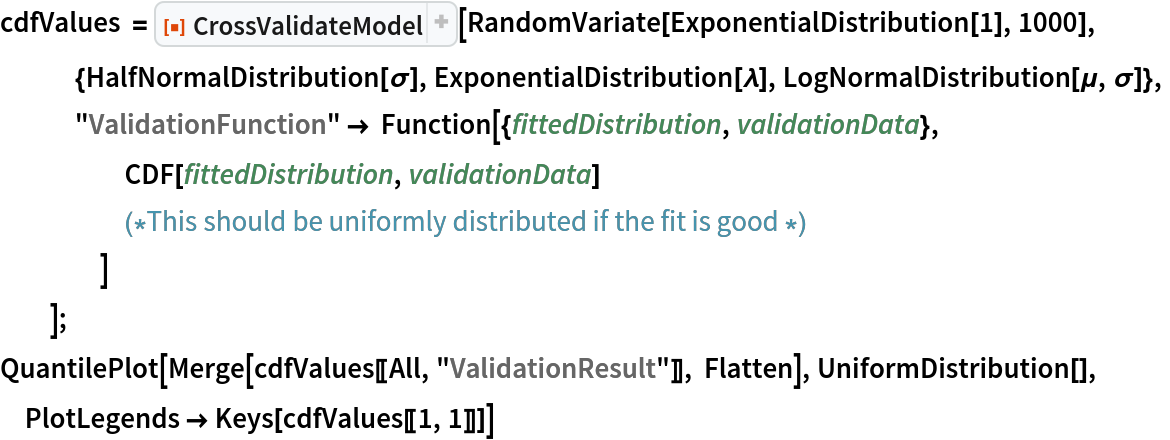Out=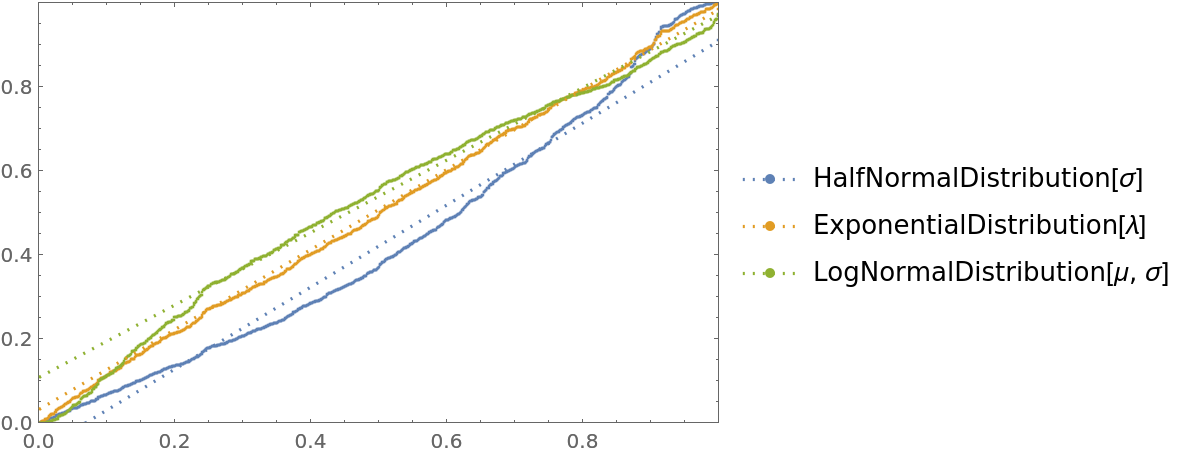For fitting functions that return a FittedModel like you can specify the validation function as "ValidationFunction" {Automatic,Function[]}. In that case, the function will be applied to the fitted values and true values in the validation set:

 In:=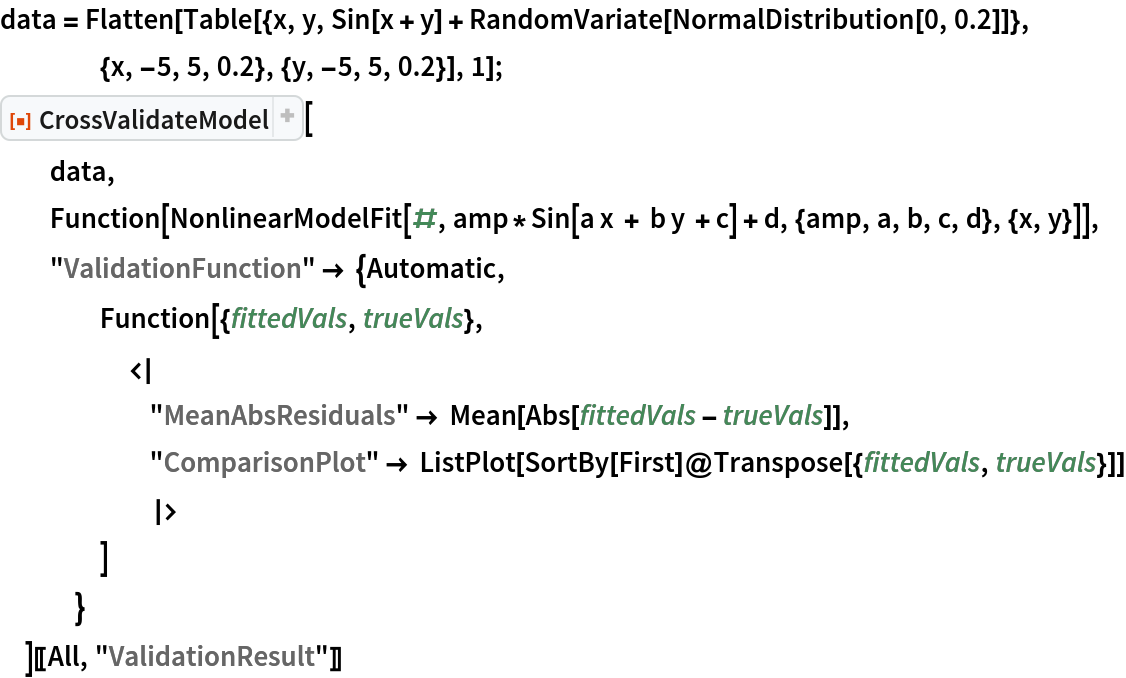Out=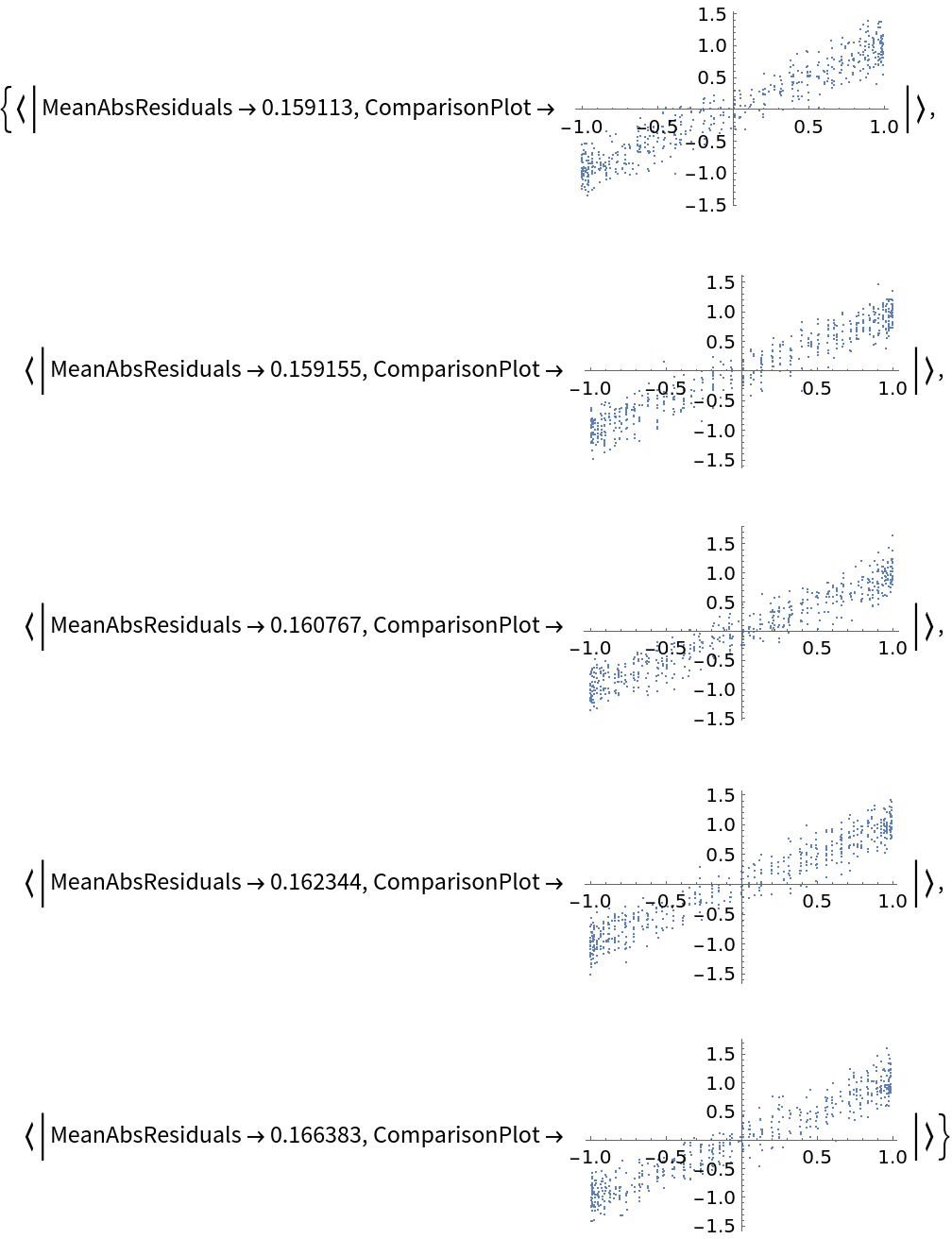For Predict and Classify, the form "ValidationFunction" {Automatic,prop} can be used to extract specific properties from PredictorMeasurements or ClassifierMeasurements. As an example, we can generate multiple prediction comparison plots and show them together in a single graph:

 In:=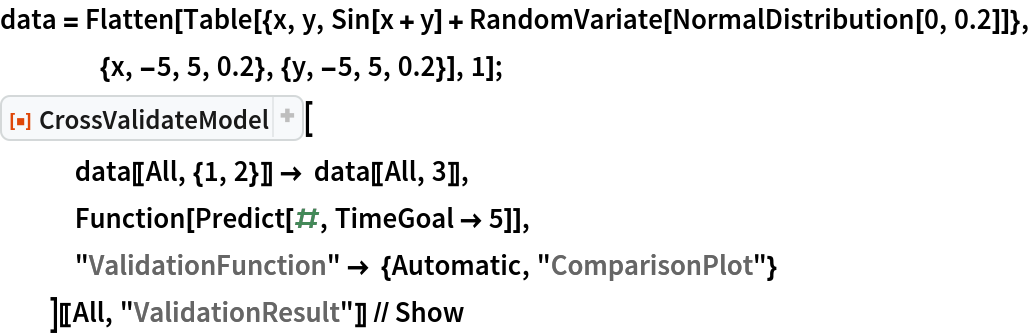Out=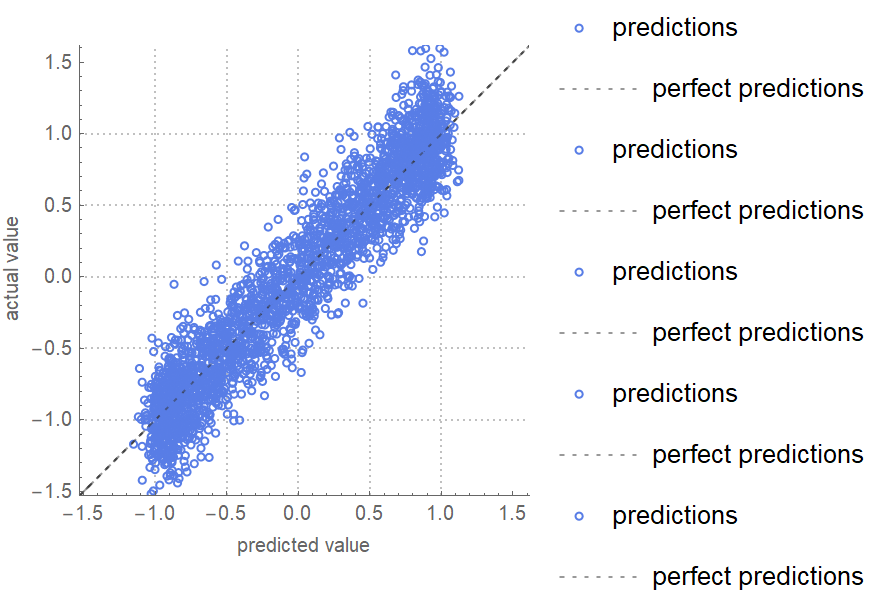For neural networks trained with NetTrain, the form "ValidationFunction" {Automatic,args} can be used to supply arguments to NetMeasurements[trainedNet,validationData,args]:

 In:=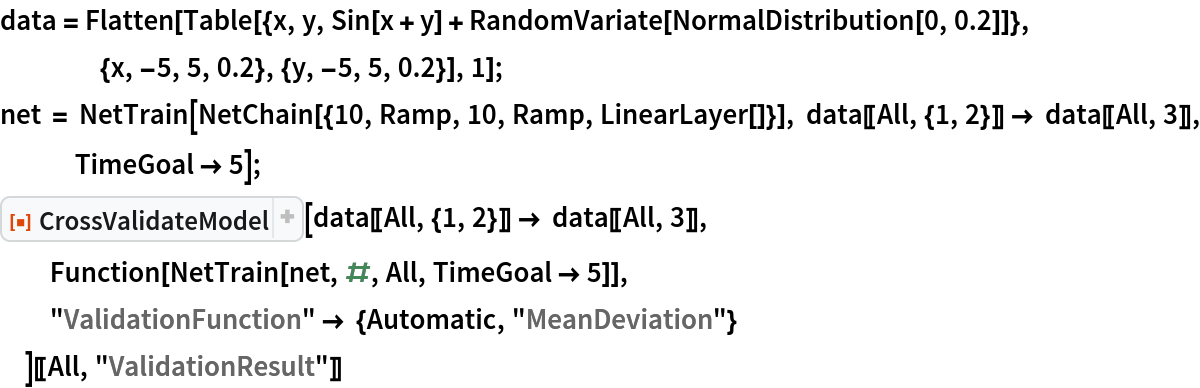Out=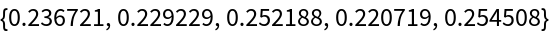With SpatialEstimate, the form "ValidationFunction" {Automatic,fun} can be used to specify a function that computes the loss from the true values, the estimated values and the standard errors of the prediction:

 In:=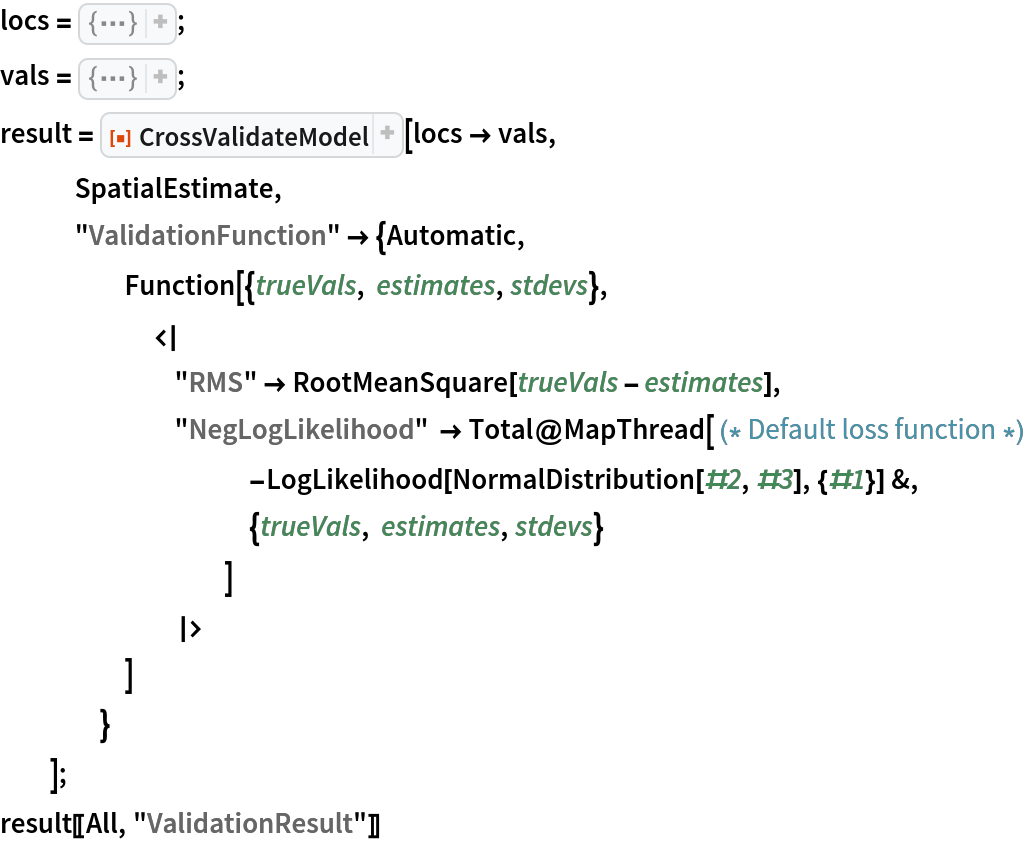Out=With "ValidationFunction" None, no validation will be performed:

 In:=Out=#### ParallelQ (1)

Use parallelization to speed up the cross-validation when doing many validation runs. Sub-options for ParallelTable can specified as "ParallelQ" {True,opts}:

 In:=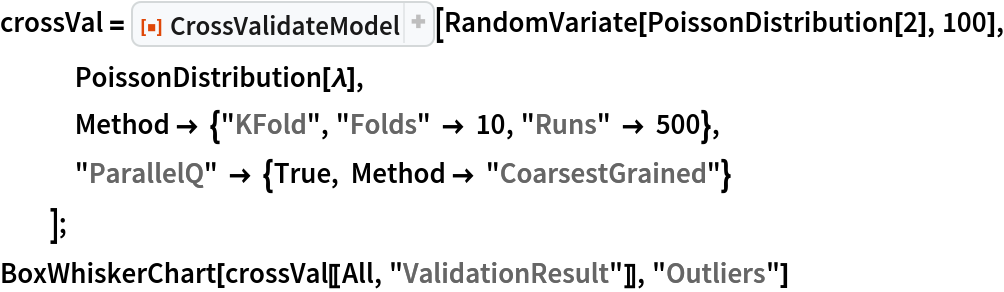Out=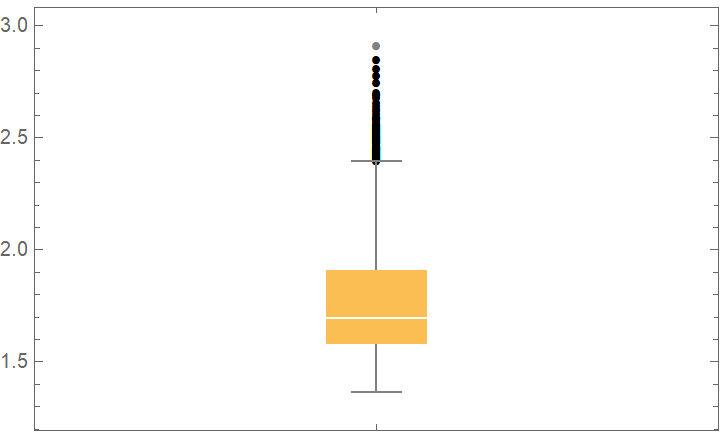### Applications (1)

Try a number of different continuous distributions to fit some ExampleData. The BoxWhiskerChart shows that the LogNormalDistribution, GammaDistribution and FrechetDistribution have the lowest losses and are therefore the best candidates:

 In:=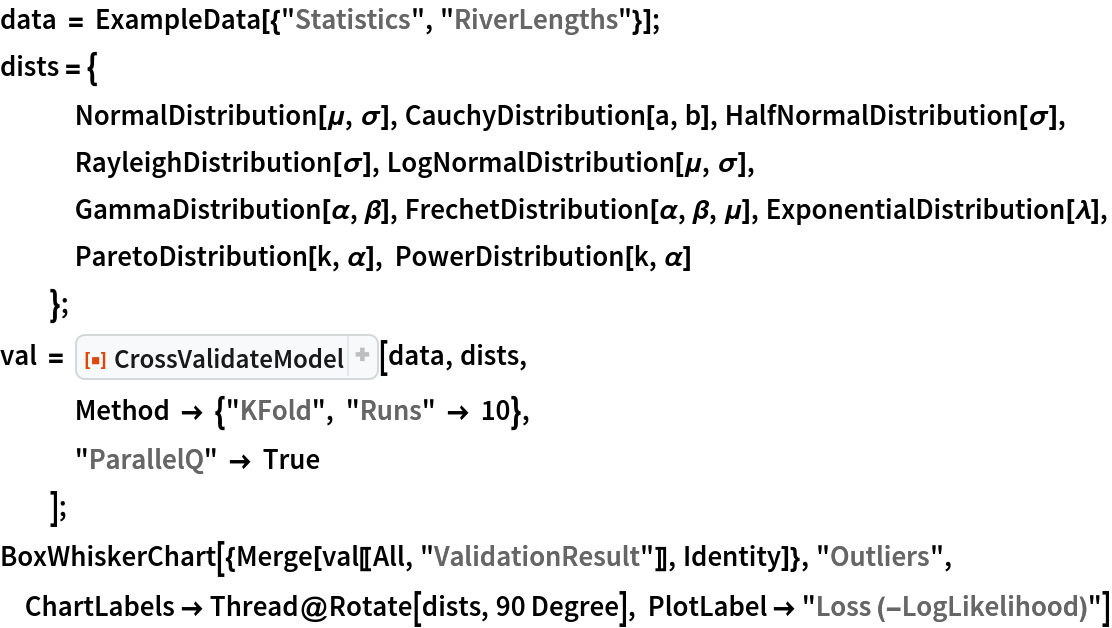Out=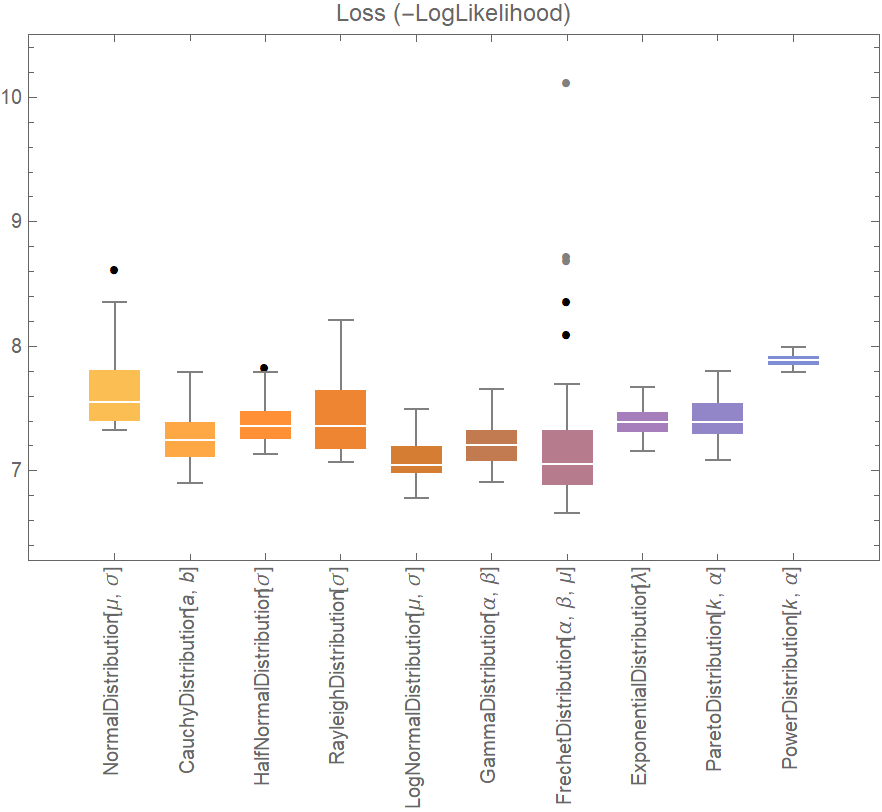### Neat Examples (1)

Compare the residual RootMeanSquare of a NonlinearModelFit and Predict:

 In:=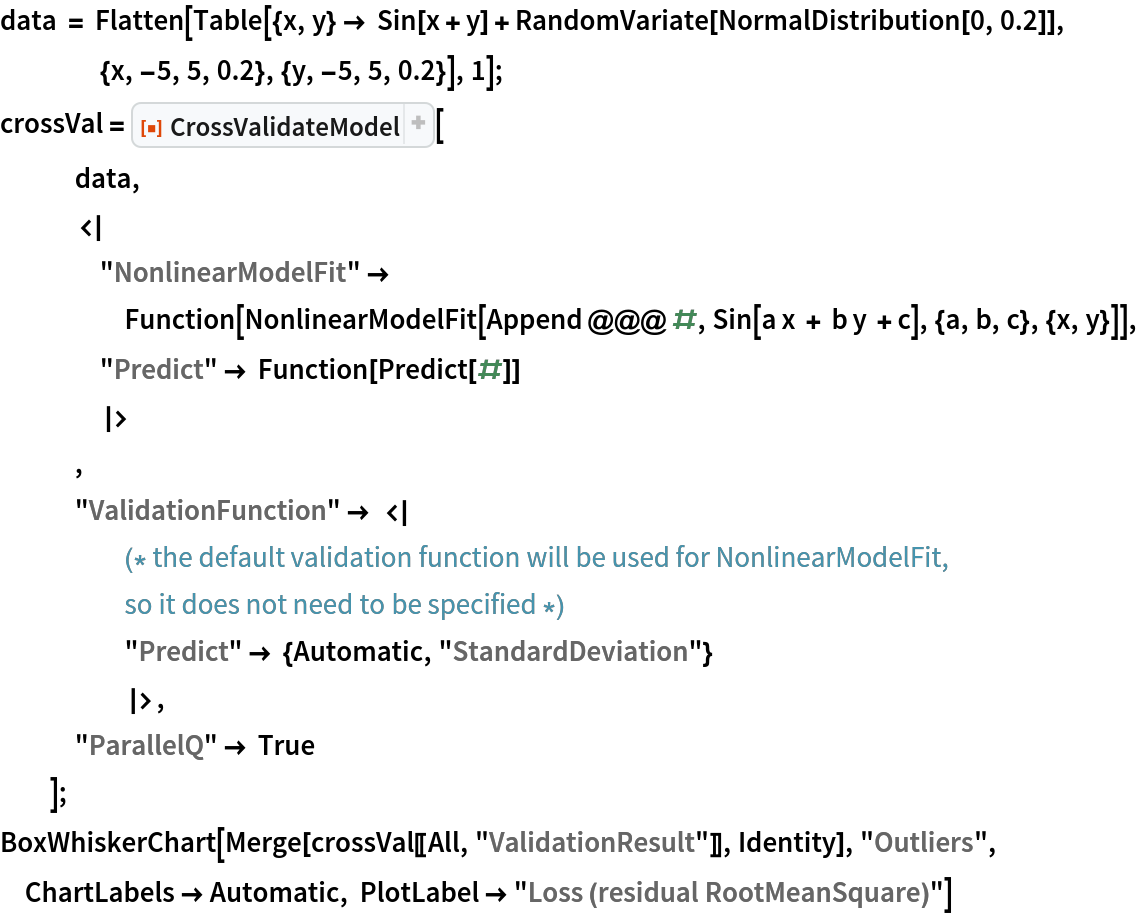Out=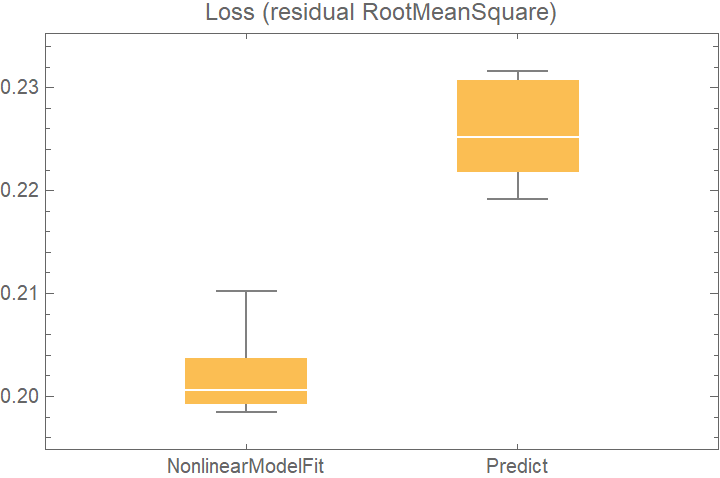Sjoerd Smit

## Version History

• 2.2.0 – 30 January 2023
• 2.1.0 – 01 March 2021
• 2.0.0 – 21 February 2020
• 1.0.0 – 19 November 2019